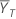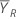# Methods and formulas for Test mean / reference mean for Equivalence Test for a 2x2 Crossover Design

The following methods and formulas are used for testing the ratio between the test mean and the reference mean.

## Ratio

The ratio, ρ, is equal to the test mean,, divided by the reference mean,, as shown below: where

## Pooled variance

The pooled variance for the reference periods, S2RR, is given by the following: The pooled variance for the test periods, S2TT, is given by the following: Let STR be defined as follows:

### Notation

TermDescription
YijkResponse for participant k during period j in sequence i (for more information, go to Methods and formulas for common concepts used in Equivalence Test for a 2x2 Crossover Design.)
niNumber of participants in sequence i

## Confidence interval for equivalence

### 100(1-α)% CI

Minitab cannot calculate the confidence interval (CI) if either of the following two conditions are not met: where S2RR represents the pooled variance for the reference periods, and S2TT represents the pooled variance for the test periods, and both S2RR and S2TTare calculated as described in the Pooled variance section, and W is given by the following: By default, Minitab calculates the 100(1 - α)% CI for the ratio as follows:

CI = [min(C, ρL), max(C, ρU)]

where: where t = t1-α,v, v = n1 + n2 – 2, and STR is calculated as described in the Pooled variance section.

### 100(1-2α)% CI

If you select the option to use the 100(1 – 2α)% CI, then the CI is given by the following:

CI = [ρL, ρU]

### One-sided intervals

For a hypothesis of Test mean / reference mean > lower limit, the 100(1 – α)% lower bound is equal to ρL.

For a hypothesis of Test mean / reference mean < upper limit, the 100(1 – α)% upper bound is equal to ρU.

### Notation

TermDescriptionMean of the reference periods (for more information, see the section on the Ratio)Mean of the test periods (for more information, see the section on the Ratio)
niNumber of participants in sequence i
vDegrees of freedom
αSignificance level for the test (alpha)
t1-α,vUpper 1 – α critical value for a t-distribution with v degrees of freedom

## T-values

Let t1 be the t-value for the hypothesis,, and let t2 be the t-value for the hypothesis,, whereis the ratio of the test mean to the reference mean for the populations. The t-values are calculated as follows: where S2RR represents the pooled variance for the reference periods, S2TT represents the pooled variance for the test periods, and STR represents the overall standard deviation. For more information, see the section on the Pooled variance.

### Notation

TermDescriptionMean of the reference periods (for more information, see the section on the Ratio)Mean of the test periods (for more information, see the section on the Ratio)
δ1Lower equivalence limit
δ2Upper equivalence limit

## P-values

The probability, PH0, for each null hypothesis is given by the following:

If, then:

H0 P-Value### Notation

TermDescription
ΛUnknown ratio of the mean of the test population to the mean of the reference population
δ1Lower equivalence limit
δ2Upper equivalence limit
vDegrees of freedom
Tt-distribution with v degrees of freedom
t1 t-value for the hypothesist2 t-value for the hypothesis###### Note

For information on how the t-values are calculated, see the section on t-values.

By using this site you agree to the use of cookies for analytics and personalized content.  Read our policy